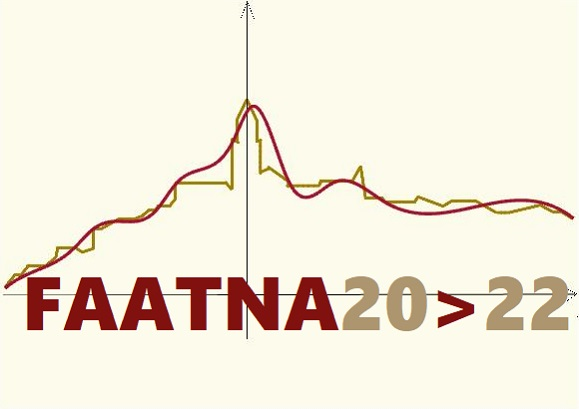# Functional Analysis, Approximation Theory and Numerical Analysis

## Matera, Italy, July 5-8, 2022

Session: Orthogonal Polynomials, Interpolation and Numerical Integration

Orthogonal Polynomials and Interpolation are in the hard core of Approximation Theory, which is the basis for the development of numerical methods used in the solution of applied problems, such as the approximate calculation of (definite) integrals. The session will connect the background theory, Orthogonal Polynomials and Interpolation, with the practical application, Numerical Integration.

Organizers:

• Sotiris Notaris, notaris@math.uoa.gr
• Miodrag Spalević, mspalevic@mas.bg.ac.rs
• Marija Stanić, stanicm@kg.ac.rs

Talks: